Guided Lessons

# Fractions and fair shares

#### Use real-world situations

This strategy focuses on how fractions are applied in real-world situations.

• Tell a story about dividing something, such as a pizza or cake, into equal parts. For example, share that you have a pizza and need to divide it into eight equal parts.• Draw a picture to represent the object you are hoping to divide.• Show how to divide the object into equal parts by drawing lines.• Count how many parts there to check your work.• Explain that one of these parts is called one eighth (⅛). Eight eighths (8/8) make one whole.• Repeat this process again, this time having your child create the equal groups.

#### Focus on equal parts

This strategy focuses on explaining the difference between equal and not equal parts.

• Draw a picture of a rectangle.• Divide the rectangle into eight not equal pieces.• Ask your child if the pieces you drew were the same or different.

• Explain that you can’t call one of the pieces you drew ⅛ because fractions refer to equal groups.

• Redraw the same rectangle.• Have your child try to divide the rectangle into eight equal pieces. Ask your child what fraction one of the pieces is.• Explain how two pieces are called two eighths, three pieces are called three eighths, etc.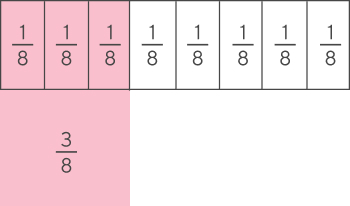#### Introduce fractional notation

This strategy focuses on introducing the proper names for each part of a fraction.

• Draw a circle.• Divide the circle into two pieces. Color in one piece. Tell your child that each piece is called one half.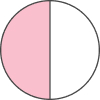• Write ½ on each peice.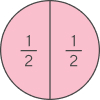• Explain that the bottom number, the denominator, shows how many equal parts the circle was divided into.

• Explain that the top number, the numerator, shows how many of those divided parts you are counting, or identifying.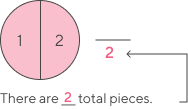• Repeat this process with other shapes, dividing them into equal parts and having your child color in different parts. And have your child write the fractions of the shapes that are colored in.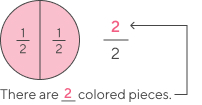#### Key words to know

• Fraction:

A number that is not a whole number, but rather a portion of a whole number

• Denominator:

The number below the line in a common fraction showing the total parts of the whole, for example 3 in ⅔.

• Numerator:

The number above the line in a common fraction showing how many of the parts of the whole are represented, for example 2 in 2/3.

#### Common Core Standards

• 3.NF

Number and Operations-Fractions